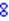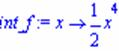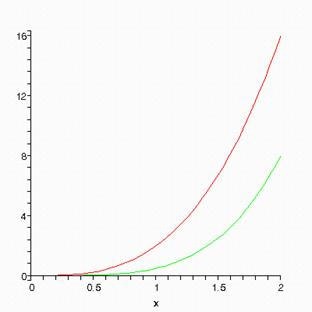# 1350 Integrals

Integrals

## Math 1350 - Integrals

MAPLE is a powerful tool in finding integrals. The MAPLE command to integrate is int.

Example 1:

integrate f(x) = 2*x^3.

> f:=x->2*x^3;> int(f(x), x);Warning: MAPLE forgets to put the constant C.

Example 2:

integrate f(x) = 2*x^3 from x=0 to x=2. Plot f(x) and its integral on the same graph.

> int(f(x), x=0..2);Name the integral int_f. Use the MAPLE command unapply to make int_f a function of x for MAPLE.

> int_f:=unapply(int(f(x), x), x);> plot([f(x), int_f(x)], x=0..2);Can you say what curve on the graph belongs to what function?

Exercises:

integrate the following function. Plot the function and its integral on the same graph.

1. f(x) = e^x from x = 0 to x = 4. Use the MAPLE command evalf(%) to get the answer as a number.
2. f(x) = sin(x) from x = p/2 to x = -p.
3. f(x) = tan(x) from x = p/2 to x = -p.
4. f(x) = tan(x/2) from x = p/2 to x = -p.
5. f(x) = tan(x)/2 from x = p/2 to x = -p.